# 3. Would it be correct to use formula that says Macid Vacid = Mbase. V base at the equivalence point of a titration...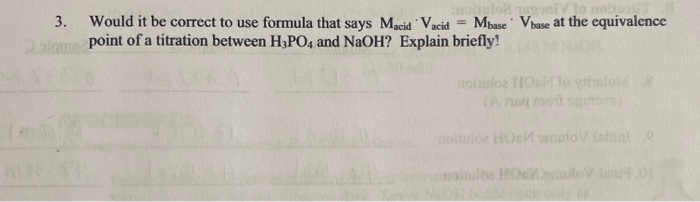3. Would it be correct to use formula that says Macid Vacid = Mbase. V base at the equivalence point of a titration between H2PO4 and NaOH? Explain briefly!

It would be correct to use formula that says Macid.Vacid=Mbase.Vbase at equivalence point of a titration between H3PO4 and NaOH.

H3PO4 is a weak acid and NaOH is a strong base. And the given formula is valid for the calculation of equivalence point of weak acid and strong base titration.

##### Add Answer of: 3. Would it be correct to use formula that says Macid Vacid = Mbase. V base at the equivalence point of a titration...
Similar Homework Help Questions
• ### 19 points) Calculate the pll at the equivalence point for the titration of 25SmL. of 0.10...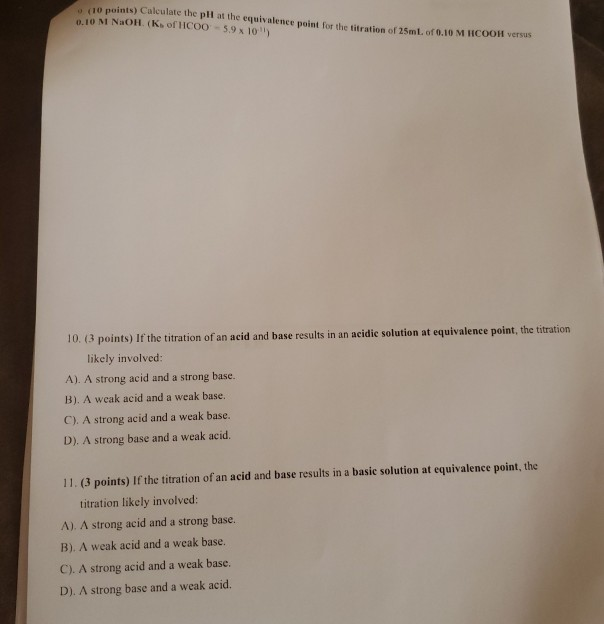19 points) Calculate the pll at the equivalence point for the titration of 25SmL. of 0.10 M HCOOH versus e.10 M NaOH. (Ks of HC0O-5.9 x 10) 10. (3 points) If the titration of an acid and base results in an acidic solution at equivalence point, the titration likely involved: A). A strong acid and a strong base. B). A weak acid and a weak base. C). A strong acid and a weak base. D). A strong base and a...

• ### compare and contrast the colorimetric determination of a titration equivalence point using an acid-base indicator versus...

compare and contrast the colorimetric determination of a titration equivalence point using an acid-base indicator versus electrochemical determination of a titration equivalence point using a pH meter. please explain pros and cons of both methods

• ### What is the pH at the equivalence point in the titration of 50.0 mL of 0.100...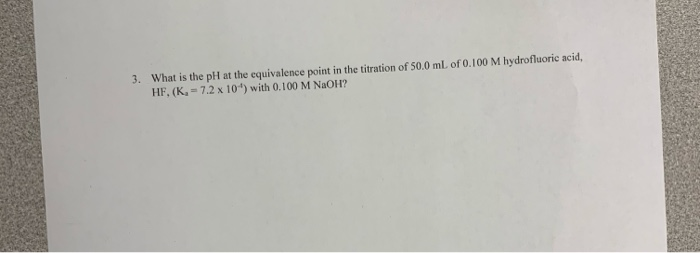What is the pH at the equivalence point in the titration of 50.0 mL of 0.100 M hydrofluoric acid, HF, (K 7.2 x 10) with 0.100 M NaOH? 3. What is the pH at the equivalence point in the titration of 50.0 mL of 0.100 M hydrofluoric acid, HF, (K 7.2 x 10) with 0.100 M NaOH? 3. What is the pH at the equivalence point in the titration of 50.0 mL of 0.100 M hydrofluoric acid, HF, (K 7.2...

• ### In the titration of the weak acid (HA) by a strong base, such as NaOH, what is meant by the equivalence point? HA)...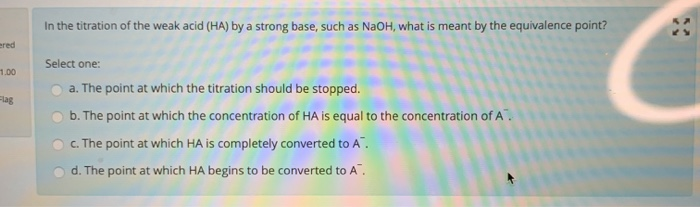In the titration of the weak acid (HA) by a strong base, such as NaOH, what is meant by the equivalence point? HA) by a strong ered 1.00 Select one: a. The point at which the titration should be stopped. b. The point at which the concentration of HA is equal to the concentration of A. Flag C. The point at which HA is completely converted to A. d. The point at which HA begins to be converted to A

• ### 1. What is the definition of an 'equivalence point' in an acid/base titration? (1 point) 2....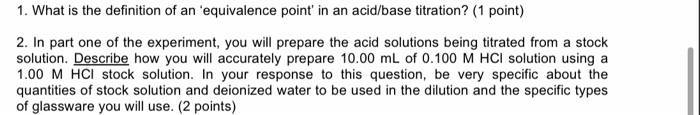1. What is the definition of an 'equivalence point' in an acid/base titration? (1 point) 2. In part one of the experiment, you will prepare the acid solutions being titrated from a stock solution. Describe how you will accurately prepare 10.00 mL of 0.100 M HCl solution using a 1.00 M HCl stock solution. In your response to this question, be very specific about the quantities of stock solution and deionized water to be used in the dilution and the...

• ### Titration and Equivalence Point

C6H5COOH(s) -- C6H5COO-(aq) + H+(aq)Ka = 6.46 x 10e-5Benzoic acid, C6H5COOH, dissociates in water as shown in the equation above. A 25.0 mL sample of an aqueous solution of pure benzoic acid is titrated usingstandardized 0.150 M NaOH.After addition of 15.0 mL of the 0.150 M NaOH, the pH of the resulting solution is 4.37.State whether the solution at the equivalence point of the titration is acidic, basic, or neutral. Explain your reasoning.Please show steps.Thank you in advance!

• ### Titration of weak acid with strong base pre-lab Answers can only be entered/graded between 2019-10-24 and...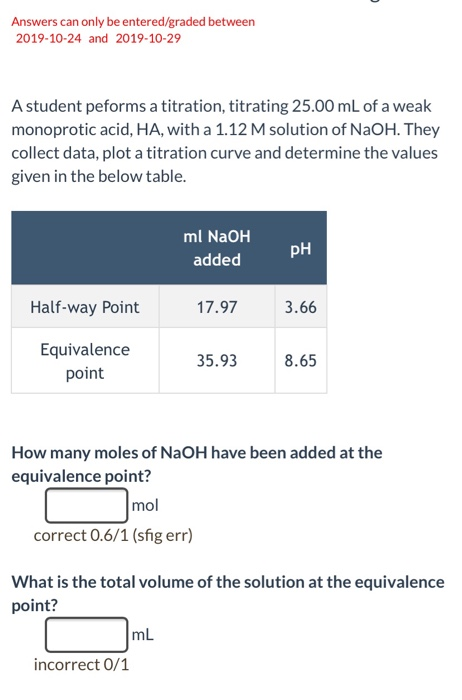Titration of weak acid with strong base pre-lab Answers can only be entered/graded between 2019-10-24 and 2019-10-29 A student peforms a titration, titrating 25.00 mL of a weak monoprotic acid, HA, with a 1.12 M solution of NaOH. They collect data, plot a titration curve and determine the values given in the below table. ml NaOH PH added Half-way Point 17.97 3.66 Equivalence point 35.938.65 How many moles of NaOH have been added at the equivalence point? r mol correct...

• ### 1. What is the definition of an ‘equivalence point’ in an acid/base titration? (1 point) 2....

1. What is the definition of an ‘equivalence point’ in an acid/base titration? (1 point) 2. In part one of the experiment, you will prepare the acid solutions being titrated from a stock solution. Describe how you will accurately prepare 10.00 mL of 0.100 M HCl solution using a 1.00 M HCl stock solution. In your response to this question, be very specific about the quantities of stock solution and deionized water to be used in the dilution and the...

• ### 5. What is the approximate pH at the equivalence point of a weak acid-strong base titration if 25 mL of an aqueous w...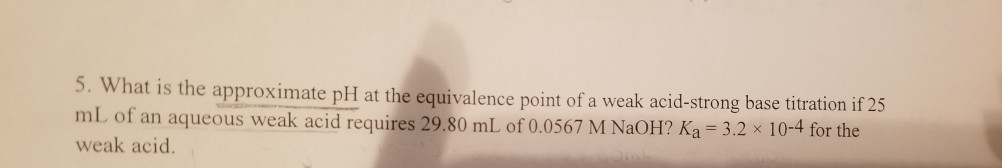5. What is the approximate pH at the equivalence point of a weak acid-strong base titration if 25 mL of an aqueous weak acid requires 29.80 mL of 0.0567 M NaOH? Ka = 3.2 x 10-4 for the weak acid.

• ### Strong acid-Strong base Titration What is the pH of the solution after 9.9 mL of 0.1...

Strong acid-Strong base Titration What is the pH of the solution after 9.9 mL of 0.1 M NaOH is added to 1.0 L of 10^-3 M HCl solution? Assume T = 25 degree C and ionic strength is negligible. Weak acid-Strong base Titration (Use Approximation equations) For a 0.001-M H_2S solution (PKa of H_2S = 7.0), titrated with a strong NaOH solution, determine the following: (a) Initial pH (b) Midpoint pH (c) Equivalence point pH Weak base-Strong acid titration(Use Approximation...

Need Online Homework Help?